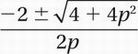# SAT Math Multiple Choice Question 770: Answer and Explanation

### Test Information

Question: 770

5. If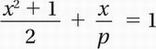, which of the following expressions gives both possible values of x, in terms of p?

• A.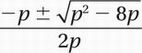• B.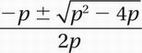• C.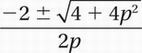• D.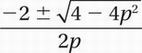Explanation:

C

Algebra (rewriting expressions) MEDIUM-HARD

Original equation: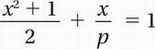Multiply by 2p (the common denominator):

p(x2 + 1) + 2x = 2p

Distribute:

px2 + p + 2x = 2p

Subtract 2p and write in descending powers of x:

px2 + 2x - p = 0

Put into quadratic formula with a = p, b = 2, and c = -p: